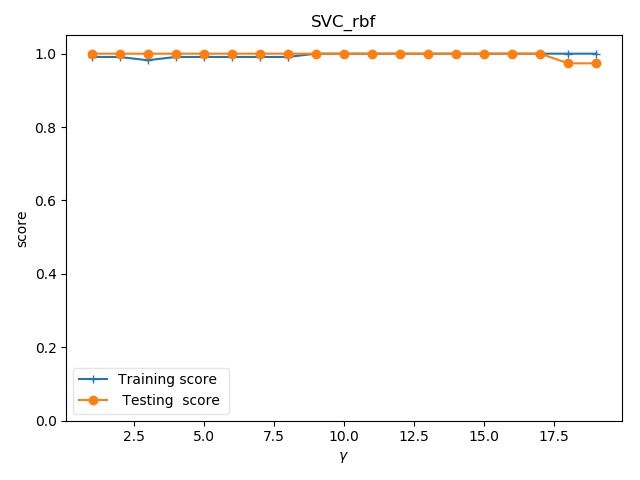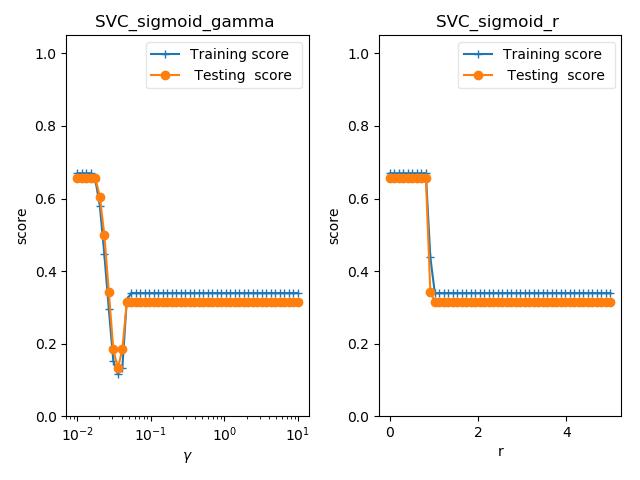• sklearnsvm基本使用

千次阅读 2020-12-11 13:08:41
此外python机器学习库scikit-learn也有svm相关算法，sklearn.svm.SVC和sklearn.svm.LinearSVC分别由libsvm和liblinear发展而来。推荐使用SVM的步骤为：将原始数据转化为SVM算法软件或包所能识别的数据格式；将数据...

SVM基本使用

SVM在解决分类问题具有良好的效果，出名的软件包有libsvm(支持多种核函数),liblinear。此外python机器学习库scikit-learn也有svm相关算法，sklearn.svm.SVC和

sklearn.svm.LinearSVC分别由libsvm和liblinear发展而来。

推荐使用SVM的步骤为：

将原始数据转化为SVM算法软件或包所能识别的数据格式；

将数据标准化；(防止样本中不同特征数值大小相差较大影响分类器性能)

不知使用什么核函数，考虑使用RBF；

利用交叉验证网格搜索寻找最优参数(C, γ)；(交叉验证防止过拟合，网格搜索在指定范围内寻找最优参数)

使用最优参数来训练模型；

测试。

下面利用scikit-learn说明上述步骤：

1 importnumpy as np2 from sklearn.svm importSVC3 from sklearn.preprocessing importStandardScaler4 from sklearn.model_selection importGridSearchCV, train_test_split5

8 假设这是鸢尾花数据,csv数据格式为：9 0,5.1,3.5,1.4,0.210 0,5.5,3.6,1.3,0.511 1,2.5,3.4,1.0,0.512 1,2.8,3.2,1.1,0.213 每一行数据第一个数字(0,1...)是标签,也即数据的类别。14 '''

15 data = np.genfromtxt(filename, delimiter=',')16 x = data[:, 1:] #数据特征

17 y = data[:, 0].astype(int) #标签

18 scaler =StandardScaler()19 x_std = scaler.fit_transform(x) #标准化

20 #将数据划分为训练集和测试集，test_size=.3表示30%的测试集

21 x_train, x_test, y_train, y_test = train_test_split(x_std, y, test_size=.3)22 returnx_train, x_test, y_train, y_test23

24

25 defsvm_c(x_train, x_test, y_train, y_test):26 #rbf核函数，设置数据权重

27 svc = SVC(kernel='rbf', class_weight='balanced',)28 c_range = np.logspace(-5, 15, 11, base=2)29 gamma_range = np.logspace(-9, 3, 13, base=2)30 #网格搜索交叉验证的参数范围，cv=3,3折交叉

31 param_grid = [{'kernel': ['rbf'], 'C': c_range, 'gamma': gamma_range}]32 grid = GridSearchCV(svc, param_grid, cv=3, n_jobs=-1)33 #训练模型

34 clf =grid.fit(x_train, y_train)35 #计算测试集精度

36 score =grid.score(x_test, y_test)37 print('精度为%s' %score)38

39 if __name__ == '__main__':

其它内容

网格搜索小技巧

网格搜索法中寻找最优参数中为寻找最优参数，网格大小如果设置范围大且步长密集的话难免耗时，但是不这样的话又可能找到的参数不是很好，针对这解决方法是，先在大范围，大步长的粗糙网格内寻找参数。在找到的参数左右在设置精细步长找寻最优参数比如：

一开始寻找范围是 C = 2−5 , 2 −3 , . . . , 2 15 and γ = 2−15 , 2 −13 , . . . , 2 3 .由此找到的最优参数是(23 , 2 −5 )；

然后设置更小一点的步长，参数范围变为21 , 2 1.25 , . . . , 2 5 and γ = 2−7 , 2 −6.75 , . . . , 2−3 在这个参数范围再寻找最优参数。

这样既可以避免一开始就使用大范围，小步长而导致分类器进行过于多的计算而导致计算时间的增加。

线性核和RBF的选择

如果训练样本的特征数量过于巨大，也许就不需要通过RBF等非线性核函数将其映射到更高的维度空间上，利用非线性核函数也并不能提高分类器的性能。利用linear核函数也可以获得足够好的结果，此外，也只需寻找一个合适参数C，但是利用RBF核函数取得与线性核函数一样的效果的话需要寻找两个合适参数(C, γ)。

分三种情况讨论：

样本数量远小于特征数量：这种情况，利用情况利用linear核效果会高于RBF核。

样本数量和特征数量一样大：线性核合适，且速度也更快。liblinear更适合

样本数量远大于特征数量： 非线性核RBF等合适。

其它文章：

展开全文• sklearnSVM函数

2019-11-20 21:29:01
sklearn SVM函数属性和参数 属性和参数

属性和参数

参数

• C: float参数 默认值为1.0
错误项的惩罚系数。C越大，即对分错样本的惩罚程度越大，因此在训练样本中准确率越高，但是泛化能力降低，也就是对测试数据的分类准确率降低。相反，减小C的话，容许训练样本中有一些误分类错误样本，泛化能力强。对于训练样本带有噪声的情况，一般采用后者，把训练样本集中错误分类的样本作为噪声。

• kernel: str参数 默认为‘rbf’
算法中采用的核函数类型，可选参数有：
‘linear’:线性核函数
‘poly’：多项式核函数
‘rbf’：径像核函数/高斯核
‘sigmod’:sigmod核函数
‘precomputed’:核矩阵

• degree: int型参数 默认为3
这个参数只对多项式核函数有用，是指多项式核函数的阶数n
如果给的核函数参数是其他核函数，则会自动忽略该参数。

• gamma: float参数 默认为auto
核函数系数，只对‘rbf’,‘poly’,‘sigmod’有效。
如果gamma为auto，代表其值为样本特征数的倒数，即1/n_features.

• coef0: float参数 默认为0.0
核函数中的独立项，只有对‘poly’和‘sigmod’核函数有用，是指其中的参数c

• probability： bool参数 默认为False
是否启用概率估计。 这必须在调用fit()之前启用，并且会fit()方法速度变慢。

• shrinking： bool参数 默认为True
是否采用启发式收缩方式

• tol: float参数 默认为1e^-3
svm停止训练的误差精度

• cache_size： float参数 默认为200
指定训练所需要的内存，以MB为单位，默认为200MB。

• class_weight： 字典类型或者‘balance’字符串。默认为None
给每个类别分别设置不同的惩罚参数C，如果没有给，则会给所有类别都给C=1，即前面参数指出的参数C.
如果给定参数‘balance’，则使用y的值自动调整与输入数据中的类频率成反比的权重。

• verbose： bool参数 默认为False
是否启用详细输出。 此设置利用libsvm中的每个进程运行时设置，如果启用，可能无法在多线程上下文中正常工作。一般情况都设为False，不用管它。

• max_iter： int参数 默认为-1
最大迭代次数，如果为-1，表示不限制

• random_state： int型参数 默认为None
伪随机数发生器的种子,在混洗数据时用于概率估计。

• fit() 方法：用于训练SVM，具体参数已经在定义SVC对象的时候给出了，这时候只需要给出数据集X和X对应的标签y即可。

• predict() 方法: 基于以上的训练，对预测样本T进行类别预测，因此只需要接收一个测试集T，该函数返回一个数组表示个测试样本的类别。

• 属性有哪些

• svc.n_support_：各类各有多少个支持向量

• svc.support_：各类的支持向量在训练样本中的索引

• svc.support_vectors_：各类所有的支持向量

示例

import matplotlib.pyplot as plt
import numpy as np
from sklearn import datasets, linear_model, model_selection, svm

X_train = iris.data
y_train = iris.target
X_train, X_test, y_train, y_test = model_selection.train_test_split(X_train, y_train, test_size=0.25, random_state=0, stratify=y_train)

cls = svm.SVC(kernel='linear')
cls.fit(X_train, y_train)
print('Coefficients:%s, intercept %s' % (cls.coef_, cls.intercept_))
print('Score: %.2f' % cls.score(X_test, y_test))

poly + degree

fig = plt.figure()

degrees = range(1, 20)
train_scores = []
test_scores = []
for degree in degrees:
cls = svm.SVC(kernel='poly', degree=degree)
cls.fit(X_train, y_train)
train_scores.append(cls.score(X_train, y_train))
test_scores.append(cls.score(X_test, y_test))

ax.plot(degrees, train_scores, label="Training score ", marker='+')
ax.plot(degrees, test_scores, label=" Testing  score ", marker='o')
ax.set_title("SVC_poly_degree ")
ax.set_xlabel("p")
ax.set_ylabel("score")
ax.set_ylim(0, 1.05)
ax.legend(loc="best", framealpha=0.5)

gammas = range(1, 20)
train_scores = []
test_scores = []
for gamma in gammas:
cls = svm.SVC(kernel='poly', gamma=gamma, degree=3)
cls.fit(X_train, y_train)
train_scores.append(cls.score(X_train, y_train))
test_scores.append(cls.score(X_test, y_test))
ax.plot(gammas, train_scores, label="Training score ", marker='+')
ax.plot(gammas, test_scores, label=" Testing  score ", marker='o')
ax.set_title("SVC_poly_gamma ")
ax.set_xlabel(r"$\gamma$")
ax.set_ylabel("score")
ax.set_ylim(0, 1.05)
ax.legend(loc="best", framealpha=0.5)

rs = range(0, 20)
train_scores = []
test_scores = []
for r in rs:
cls = svm.SVC(kernel='poly', gamma=10, degree=3, coef0=r)
cls.fit(X_train, y_train)
train_scores.append(cls.score(X_train, y_train))
test_scores.append(cls.score(X_test, y_test))
ax.plot(rs, train_scores, label="Training score ", marker='+')
ax.plot(rs, test_scores, label=" Testing  score ", marker='o')
ax.set_title("SVC_poly_r ")
ax.set_xlabel(r"r")
ax.set_ylabel("score")
ax.set_ylim(0, 1.05)
ax.legend(loc="best", framealpha=0.5)
plt.show()rbf + gamma

gammas = range(1, 20)
train_scores = []
test_scores = []
for gamma in gammas:
cls = svm.SVC(kernel='rbf', gamma=gamma)
cls.fit(X_train, y_train)
train_scores.append(cls.score(X_train, y_train))
test_scores.append(cls.score(X_test, y_test))
fig = plt.figure()
ax.plot(gammas, train_scores, label="Training score ", marker='+')
ax.plot(gammas, test_scores, label=" Testing  score ", marker='o')
ax.set_title("SVC_rbf")
ax.set_xlabel(r"$\gamma$")
ax.set_ylabel("score")
ax.set_ylim(0, 1.05)
ax.legend(loc="best", framealpha=0.5)
plt.show()sigmoid + gamma + coef

fig = plt.figure()

gammas = np.logspace(-2, 1)
train_scores = []
test_scores = []

for gamma in gammas:
cls = svm.SVC(kernel='sigmoid', gamma=gamma, coef0=0)
cls.fit(X_train, y_train)
train_scores.append(cls.score(X_train, y_train))
test_scores.append(cls.score(X_test, y_test))
ax.plot(gammas, train_scores, label="Training score ", marker='+')
ax.plot(gammas, test_scores, label=" Testing  score ", marker='o')
ax.set_title("SVC_sigmoid_gamma ")
ax.set_xscale("log")
ax.set_xlabel(r"$\gamma$")
ax.set_ylabel("score")
ax.set_ylim(0, 1.05)
ax.legend(loc="best", framealpha=0.5)

rs = np.linspace(0, 5)
train_scores = []
test_scores = []

for r in rs:
cls = svm.SVC(kernel='sigmoid', coef0=r, gamma=0.01)
cls.fit(X_train, y_train)
train_scores.append(cls.score(X_train, y_train))
test_scores.append(cls.score(X_test, y_test))
ax.plot(rs, train_scores, label="Training score ", marker='+')
ax.plot(rs, test_scores, label=" Testing  score ", marker='o')
ax.set_title("SVC_sigmoid_r ")
ax.set_xlabel(r"r")
ax.set_ylabel("score")
ax.set_ylim(0, 1.05)
ax.legend(loc="best", framealpha=0.5)
plt.show()展开全文sklearn SVM 机器学习
• 使用sklearn简单进行SVM参数优选

万次阅读 多人点赞 2018-03-22 16:56:50
SVM简单回顾 支持向量机（SVM）方法建立在统计学VC维和结构风险最小化原则上，试图寻找到一个具有最大分类间隔的超平面，支持向量（Support Vector）是支持向量机训练的结果，在进行分类或者回归时也只依赖支持向量...

SVM简单回顾

支持向量机（SVM）方法建立在统计学VC维和结构风险最小化原则上，试图寻找到一个具有最大分类间隔的超平面，支持向量（Support Vector）是支持向量机训练的结果，在进行分类或者回归时也只依赖支持向量。因此SVM具有良好的鲁棒性，对未知数据拥有很强的泛化能力，特别是在数据量较少的情况下，相较其他传统机器学习算法具有更优的性能。
对于样本数据线性不可分的情况，通常采用核方法，将原始空间中的样本数据映射到一个更高维的特征空间，在该高维空间中样本数据线性可分。常用的核函数有线性核函数（Linear Kernel）、多项式核函数（Polynomial Kernel）、高斯核函数（RBF Kernel）和Sigmoid核函数（Sigmoid Kernel）。

名称表达式参数
线性核 k ( u , v ) = u T v + c k(u,v)=u^Tv+c
多项式核 k ( u , v ) = ( r ∗ u T v + c ) d k(u,v)=(r*u^Tv+c)^d c， d ≥ 1 d\geq1 为多项式次数
高斯核\$k(u,v)=exp(-\frac{
Sigmoid核 k ( u , v ) = t a n h ( r ∗ u T v + c ) k(u,v)=tanh(r*u^Tv+c) tanh为双曲正切函数

使用SVM作为模型时，通常采用如下流程：

1. 对样本数据进行归一化
2. 应用核函数对样本进行映射
3. 用cross-validation和grid-search对超参数进行优选
4. 用最优参数训练得到模型
5. 测试

在第二步中最长采用和核函数是RBF核函数和线性核函数，在样本线性可分时，线性核函数效果要比RBF核函数好，有如下指导规则：

1. 如果Feature的数量很大，甚至和样本数量差不多时，往往线性可分，这时选用LR或者线性核SVM；
2. 如果Feature的数量很小，样本数量正常，不算多也不算少，这时选用RBF核SVM；
3. 如果Feature的数量很小，而样本的数量很大，这时手动添加一些Feature，使得线性可分，然后选用LR或者线性核SVM。

Libsvm参数

Libsvm是一个简单的、容易使用的、高效的实现了SVM分类和回归的工具包。Libsvm可以从该链接获取
当使用svm-train时，主要涉及以下参数：
-s svm_type : set type of SVM (default 0)
0 – C-SVC (multi-class classification)
1 – nu-SVC (multi-class classification)
2 – one-class SVM
3 – epsilon-SVR (regression)
4 – nu-SVR (regression)

-t kernel_type : set type of kernel function (default 2)
0 – linear: u’v
1 – polynomial: (gamma
u’v + coef0)^degree
2 – radial basis function: exp(-gamma
|u-v|^2)
3 – sigmoid: tanh(gamma*u’*v + coef0)

-d degree : set degree in kernel function (default 3)，设置多项式核的degree值
-g gamma : set gamma in kernel function (default 1/num_features)，多项式/RBF/Sigmoid核的gamma值
-r coef0 : set coef0 in kernel function (default 0)，设置多项式/Sigmoid核的coef0值
-c cost : set the parameter C of C-SVC, epsilon-SVR, and nu-SVR (default 1)，设置惩罚函数的力度
-n nu : set the parameter nu of nu-SVC, one-class SVM, and nu-SVR (default 0.5)，设置nu-SVC/one-class SVM/nu-SVR的nu值
-p epsilon : set the epsilon in loss function of epsilon-SVR (default 0.1)，设置epsilon-SVR的损失函数中epsilon值
-m cachesize : set cache memory size in MB (default 100)，设置cache内存的大小，单位MB
-e epsilon : set tolerance of termination criterion (default 0.001)，设置终止的判据
-h shrinking : whether to use the shrinking heuristics, 0 or 1 (default 1)，是否允许使用启发式
-b probability_estimates : whether to train a SVC or SVR model for probability estimates, 0 or 1 (default 0)，是否进行概率估计
-wi weight : set the parameter C of class i to weightC, for C-SVC (default 1)，设置C-SVC中第i类的参数C为weightC
-v n: n-fold cross validation mode，设置交叉检验
-q : quiet mode (no outputs)

对于RBF核函数，其中比较重要的参数有c，g。其中c表示对误差的惩罚程度，c越大，表示对误差的惩罚程度越大，模型对样本数据的学习更精确，也因此容易过拟合；反之，c越小，对误差的惩罚程度越小，可能欠拟合。g对应RBF核中的gamma值，gamma越大， σ \sigma 越小，使得高斯分布又高又瘦，造成模型只能作用于支持向量附近，也就是过拟合；反之，gamma越小， σ \sigma 越大，高斯分布会过于平滑，在训练集上分类效果不佳，也就是欠拟合。
最佳c，g组合的确定可以采用最简单的网格搜索方法，sklearn中提供了相应的方法GridSearchCV：

class sklearn.model_selection.GridSearchCV(estimator, param_grid, scoring=None, fit_params=None, n_jobs=1, iid=True, refit=True, cv=None, verbose=0, pre_dispatch='2*n_jobs', error_score='raise', return_train_score=True)

比较重要的参数有：
estimator：使用的模型
param_grid：参数列表，如下展示了分别使用RBF核函数与线性核函数时参数选择

parameters = [
{
'C': [1, 3, 5, 7, 9, 11, 13, 15, 17, 19],
'gamma': [0.00001, 0.0001, 0.001, 0.1, 1, 10, 100, 1000],
'kernel': ['rbf']
}，
{
'C': [1, 3, 5, 7, 9, 11, 13, 15, 17, 19],
'kernel': ['linear']
}
]

scoring：评价指标，默认采用estimator的score函数
cv：交叉验证参数，默认为3，使用3折交叉验证
n_jobs：并行数

之后调用GridSearchCV对象的fit()方法在训练数据集上运行网格搜索，选出最优参数，然后在测试集上测试模型。GridSearchCV对象还包括如下常用属性：
grid.scores：给出不同参数组合下的评价结果
grid.best_params_：最佳结果的参数组合
grid.best_score_：最佳评价得分
grid.best_estimator_：最佳模型
完整的例子如下：

from sklearn.svm import SVC
from sklearn.model_selection import GridSearchCV

svc = SVC()
parameters = [
{
'C': [1, 3, 5, 7, 9, 11, 13, 15, 17, 19],
'gamma': [0.00001, 0.0001, 0.001, 0.1, 1, 10, 100, 1000],
'kernel': ['rbf']
}，
{
'C': [1, 3, 5, 7, 9, 11, 13, 15, 17, 19],
'kernel': ['linear']
}
]
clf = GridSearchCV(svc, parameters, cv=5, n_jobs=8)
clf.fit(train_data, train_data_tag)
print(clf.best_params_)
best_model = clf.best_estimator_
best_model.predict(test_data)

当然随着数据量的增大，GridSearchCV的效率将变得非常低，可以采用一些其他更加高级的调优方法。

展开全文sklearn svm
• 1.SVM简介 支持向量机（Support Vector Machine, SVM）是一类按监督学习（supervised learning）方式对数据进行二元分类（binary classification）的广义线性分类器（generalized linear classifier），其决策边界是...

1.SVM简介

支持向量机（Support Vector Machine, SVM）是一类按监督学习（supervised learning）方式对数据进行二元分类（binary classification）的广义线性分类器（generalized linear classifier），其决策边界是对学习样本求解的最大边距超平面（maximum-margin hyperplane）。
SVM的基本思想是：找到集合边缘上的若干数据（称为支持向量（Support Vector）），用这些点找出一个平面（称为决策面），使得支持向量到该平面的距离最大。

2.SVM参数意义

在sklearn中，封装好各种机器学习的库，其中就包含SVM算法，其调用如下：

import sklearn.svm as svm
model = svm.SVC(C=1.0,
kernel='rbf',
degree=3,
gamma='auto',
coef0=0.0,
shrinking=True,
probability=False,
tol=0.001,
cache_size=200, c
lass_weight=None,
verbose=False,
max_iter=-1,
decision_function_shape=None,
random_state=None)

参数说明：
C：SVC的惩罚参数，默认值是1.0；C越大，对误分类的惩罚增大，趋向于对训练集完全分对的情况，这样对训练集测试时准确率很高，但泛化能力弱C值小，对误分类的惩罚减小，允许容错，将他们当成噪声点，泛化能力较强

关于松弛变量，我这边要解释下：
在大多数情况下，数据并不是完美的线性可分数据，可能会存在少量的点出现在分类超平面的另外一侧。我们希望尽量保证将这些点进行正确分类，同时又保证分类面与两类样本点有足够大的几何间隔。在这种情况下，我们为每一个样本点加上一个松弛变量，允许有小的误差存在。在加入松弛变量后，我们还要在目标函数中加入相应的惩罚参数C，对这个松弛变量起到一个监督克制的作用。两者的关系，有点类似道家的阴阳制衡的关系，此消彼长。

kernel ：核函数，默认是rbf，可以是‘linear’, ‘poly’, ‘rbf’, ‘sigmoid’, ‘precomputed’
linear：线性分类器（C越大分类效果越好，但有可能会过拟合（default C=1））
poly：多项式分类器
rbf：高斯模型分类器（gamma值越小，分类界面越连续；gamma值越大，分类界面越“散”，分类效果越好，但有可能会过拟合。）
sigmoid：sigmoid核函数
具体可以参考：https://zhuanlan.zhihu.com/p/157722218
degree ：多项式poly函数的维度，默认是3，选择其他核函数时会被忽略。
gamma ： ‘rbf’,‘poly’ 和‘sigmoid’的核函数参数。默认是’auto’。 如果gamma是’auto’，那么实际系数是1 / n_features。
coef0 ：核函数中的独立项。 它只在’poly’和’sigmoid’中很重要。
probability ：是否启用概率估计。 必须在调用fit之前启用它，并且会减慢该方法的速度。默认为False
shrinking ：是否采用shrinking heuristic方法(收缩启发式)，默认为true
tol ：停止训练的误差值大小，默认为1e-3
cache_size ：核函数cache缓存大小，默认为200
class_weight ：类别的权重，字典形式传递。设置第几类的参数C为weight*C(C-SVC中的C)
verbose ：允许冗余输出
max_iter ：最大迭代次数。-1为无限制。
decision_function_shape ：‘ovo’, ‘ovr’ or None, default=ovr
关于‘ovo’, ‘ovr’的解释：
一对多法（one-versus-rest,简称OVR SVMs）：训练时依次把某个类别的样本归为一类,其他剩余的样本归为另一类，这样k个类别的样本就构造出了k个SVM。分类时将未知样本分类为具有最大分类函数值的那类。
一对一法（one-versus-one,简称OVO SVMs或者pairwise）：其做法是在任意两类样本之间设计一个SVM，因此k个类别的样本就需要设计k(k-1)/2个SVM。当对一个未知样本进行分类时，最后得票最多的类别即为该未知样本的类别。
详细讲解，可以参考这篇博客：https://blog.csdn.net/xfchen2/article/details/79621396
random_state ：数据洗牌时的种子值，int值,default=None
在随机数据混洗时使用的伪随机数生成器的种子。 如果是int，则random_state是随机数生成器使用的种子; 如果是RandomState实例，则random_state是随机数生成器; 如果为None，则随机数生成器是np.random使用的RandomState实例。
个人认为最重要的参数有：C、kernel、degree、gamma、coef0、decision_function_shape。

3.sklearn中SVM接口调用

# 建立模型
model = svm.SVC(C=10, kernel='linear')

# 训练模型,x为训练集， y为标签
model.fit(x, y)

# 预测,test为测试集
pre_y = model.predict(test)

# 预测结果数据
n_Support_vector = clf.n_support_  # 支持向量个数
sv_idx = clf.support_  # 支持向量索引
Support_vector = clf.support_vectors_   # 支持向量
w = clf.coef_  # 权重矩阵W
b = clf.intercept_  # 偏置矩阵b

4.代码实现

运行环境：pycharm、scikit-learn 0.23.2

import numpy as np
import joblib
from sklearn import svm
import matplotlib.pyplot as plt
x = [[1, 2], [4, 5], [18, 9], [12, 6], [2, 3], [13, 18]]
x = np.array(x)
y = [1, 1, 0, 0, 1, 0]
y = np.array(y)
# 训练模型
model = svm.SVC(C=10, kernel='linear')
model.fit(x, y)

# 预测
a = [[8, 6]]
a_pre = model.predict(a)
print("a_pre:", a_pre)
# 对应的支持向量
Support_vector = model.support_vectors_
print("Support_vector:", Support_vector)
# 线性分类对应的参数
w = model.coef_
print("w:", w)
b = model.intercept_
print("b:", b)
# 训练集散点图
plt.scatter(x[:, 0], x[:, 1])

if w[0, 1] != 0:
xx = np.arange(0, 20, 0.1)
# 最佳分类线
yy = -w[0, 0]/w[0, 1] * xx - b/w[0, 1]
plt.scatter(xx, yy, s=4)
# 支持向量
b1 = Support_vector[0, 1] + w[0, 0]/w[0, 1] * Support_vector[0, 0]
b2 = Support_vector[1, 1] + w[0, 0]/w[0, 1] * Support_vector[1, 0]
yy1 = -w[0, 0] / w[0, 1] * xx + b1
plt.scatter(xx, yy1, s=4)
yy2 = -w[0, 0] / w[0, 1] * xx + b2
plt.scatter(xx, yy2, s=4)
else:
xx = np.ones(100) * (-b) / w[0, 0]
yy = np.arange(0, 10, 0.1)
plt.scatter(xx, yy)
plt.show()

展开全文机器学习
• sklearn中的svm参数介绍

万次阅读 2017-04-24 23:52:11
svmsklearn中一个关于支持向量机的包，比较常用，在使用过程中若是不熟悉各个参数的意义，总以默认参数进行机器学习，则不能做到最优化使用SVM，这就是一个较为遗憾的事情了。为了加深理解和方便调用，根据现有...svm 机器学习 参数选择 参数优化 python
• SKlearn中的svm超参数总结

万次阅读 2018-05-23 11:54:27
SKlearn中好多机器学习模型已经做好了，使用的时候直接调用就可以，俗称“调包侠”，我觉得挺有意思，这样大大降低了机器学习的门槛，最近几天一直在使用svm训练模型，其中数据样本不均衡以及打分一直上不去，特征...svm
• 关于实战部分可以参考这篇文章（有源码，可直接运行）：【Sklearn】【实战】【SVM】乳腺癌检测，模拟线上部署（1） 这里是官方说明文档传送门：sklearn.svm.SVC 2. 简介 SVC为Support Vector Classification的简写...python sklearn 机器学习
• 在调用sklearnSVM时，如果设置verbose=True,模型训练结束后会显示一些训练过程的说明信息，如下(以下是OCSVM的返回结果)： * optimization finished, # iter = 32 obj = 192.154077, rho = 19.244379 nSV = 26, ...
• scikit-learn支持向量机算法库使用小结之前通过一个系列对支持向量机(以下简称SVM)算法的原理做了一个总结，本文从实践的角度对scikit-learnSVM算法库的使用做一个小结。scikit-learnSVM算法库封装了libsvm和...
• sklearnSVM使用

万次阅读 多人点赞 2018-01-17 17:49:12svm python 机器学习
• from sklearn.svm import SVC clf = SVC(kernel='rbf')#调参 clf.fit(X, y)#训练 print(clf.fit(X, y))#输出参数设置 p = 1#正确分类的个数 for i in range(len(test_dataset)):#循环检测测试数据分类成功的个数 ...SVM 二分类 SKlearn
• sklearn中svm详解

千次阅读 2019-06-16 14:56:26
1、SVM函数原型 SVC(C=1.0, cache_size=200, class_weight=None, coef0=0.0, decision_function_shape=None, degree=3, gamma=‘auto’, kernel=‘rbf’, max_iter=-1, probability=False, random_state=None, ...机器学习 python
• 【机器学习】sklearn-SVM调参

千次阅读 2019-08-06 10:36:03
SVM参数解释 （1）C: 目标函数的惩罚系数C，用来平衡分类间隔margin和错分样本的，default C = 1.0； （2）kernel：参数选择有RBF, Linear, Poly, Sigmoid, 默认的是"RBF"; （3）degree：if you choose 'Poly' in...
• SVM基本概要与sklearn.svm.svc 参数说明

万次阅读 多人点赞 2017-07-12 13:28:53
SVM的基本概念先介绍一些简单的基本概念：分隔超平面：将数据集分割开来的直线叫做分隔超平面。超平面：如果数据集是N维的，那么就需要N-1维的某对象来对数据进行分割。该对象叫做超平面，也就是分类的决策边界。...svm
• Sklearn参数详解—SVM

千次阅读 2018-05-26 14:42:30
总第108篇本篇主要讲讲SklearnSVMSVM主要有LinearSVC、NuSVC和SVC三种方法，我们将具体介绍这三种分类方法都有哪些参数值以及不同参数值的含义。在开始看本篇前...
• 1.SVM简介 　SVM方法建立在统计学VC维和结构风险最小化原则上，既可以用于分类（二/多分类）、也可用于回归和异常值检测。SVM具有良好的鲁棒性，对未知数据拥有很强的泛化能力，特别是在数据量较少的情况下，相较...
• 利用 sklearn 实现SVM，及其人脸分类小实例 利用sklearn 生成数据 import numpy as np import matplotlib.pyplot as plt from scipy import stats # 利用sklearn 随机生成数据 from sklearn.datasets.samples_...
• SVC中计算点到超平面的距离使用svc.decision_function(data)， 计算概率的公式是1/(1+exp(A*距离+B) 可以直接使用svc.predict_proba()或者 1/(1+np.exp(svc.probA_*svc.decision_function(data)+svc.probB_))，结果...机器学习 逻辑回归 svm 支持向量机
• sklearnsvm.LinearSVC的参数说明

万次阅读 多人点赞 2018-07-27 14:29:11
Sklearn.svm.LinearSVC参数说明 与参数kernel ='linear'的SVC类似，但是以liblinear而不是libsvm的形式实现，因此它在惩罚和损失函数的选择方面具有更大的灵活性，并且应该更好地扩展到大量样本。 此类支持密集和...
• sklearnSVM算法自动调优

千次阅读 2017-05-19 15:03:41
#导入，处理数据集import pandas as pd ...from sklearn import svm from sklearn.model_selection import GridSearchCV from sklearn.model_selection import train_test_splitdataset1=pd.read_excel("httpsvm 机器学习
• sklearnsvm实现文本分类 入门

千次阅读 2017-09-22 11:14:56
正在学习sklearn , 实验室项目需要实现一些文本的分类的功能。 sklearn提供了许多机器学习方面使用的到的写好的工具。其中也包括分类器。sklearn在这里不作介绍。有官网，有博客，也正在学习中最开始是参照着这片...svm 机器学习
• python SVM 案例，sklearn.svm.SVC 参数说明

万次阅读 多人点赞 2018-03-09 17:02:59
sklearn.svm.SVC 参数说明 经常用到sklearn中的SVC函数，这里把文档中的参数翻译了一些，以备不时之需。 本身这个函数也是基于libsvm实现的，所以在参数设置上有很多相似的地方。（PS: libsvm中的二次规划问题的...python svm svm sklearn svm.SVC SVM参数说明
• 最近在学习机器学习中的SVM，其中SVM中的SVC的一个属性coef_有些困惑，感谢下面的文章，所以转载收藏一下(侵权联系必删)： 文章链接：... 文章题目：sklearn中的svm参数介绍 文章作者：晓东邪 ...机器学习
• 大家都知道，SVM如果在调参比较好的情况下，可以达到很好的分类效果，不过SVM也确实参数比较多，例如在这里介绍的：https://blog.csdn.net/xiaodongxiexie/article/details/70667101也有些朋友对调参过程做了比较...
• svm.clf和scaler有几个参数需要说明： #权重系数w svm_clf1.coef_ #截距 svm_clf1.intercept_ #均值 scaler.mean_ #缩放比例 scaler.scale_  除了使用 LinearSVC类 ，还可以选择SVC类，使用 SVC（kernel=...SVM sklearn
• 由于SVM是需要不断的寻找可以最优区分开数据之间的超平面直到计算出的结果收敛为止，但由于数据的变化，例如区分高矮的准则是，超过180cm就是高，否则就不是的这种，当你给出身高的数据时，数据间具有明显的线性关系...SVM 机器学习 python
• 在python sklearn使用 SVM做分类

万次阅读 多人点赞 2018-04-19 00:37:10
sklearnSVM SVC（C-Support Vector Classification）实现基于libsvm,台湾大学林智仁教授团队开发的一个库。支持多分类。   1. SVM二分类   &gt;&gt;&gt; import numpy as np &gt;&...SVM sklearn python
• 使用sklearn实现svm分类任务

千次阅读 2020-03-29 14:00:36
判断是否幸福？ 首先导入相关包： import numpy as np import pandas as pd import matplotlib.pyplot as plt ...from sklearn.svm import SVC, LinearSVC from sklearn.model_selection import train_test_spli...机器学习 python
• 在前面的一篇文章我们给出了使用Sklearn 中的SVM进行分类时如何使用留一法来进行分类。 python基于sklearnSVM和留一法（LOOCV）进行二分类 如何在使用留一法时绘制ROC曲线和AUC 什么是ROC曲线和？这个可以参考如下...python 算法
• Sklearn.svm.LinearSVC(penalty=’l2’, loss=’squared_hinge’, dual=True, tol=0.0001, C=1.0, multi_class=’ovr’,fit_intercept=True, intercept_scaling=1, class_weight=None, verbose=0, random_state=None......